x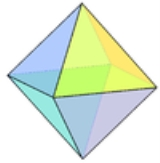Square pyramidEncyclopedia
In geometry
Geometry
Geometry arose as the field of knowledge dealing with spatial relationships. Geometry was one of the two fields of pre-modern mathematics, the other being the study of numbers ....

, a square pyramid is a pyramid
Pyramid (geometry)
In geometry, a pyramid is a polyhedron formed by connecting a polygonal base and a point, called the apex. Each base edge and apex form a triangle. It is a conic solid with polygonal base....

having a square
Square (geometry)
In geometry, a square is a regular quadrilateral. This means that it has four equal sides and four equal angles...

base. If the apex
Apex (geometry)
In geometry, an apex is the vertex which is in some sense the highest of the figure to which it belongs.*In an isosceles triangle, the apex is the vertex where the two sides of equal length meet, opposite the unequal third side....

is perpendicularly above the center of the square, it will have C4v symmetry.

## Johnson solid (J1)

If the sides are all equilateral triangles, the pyramid is one of the Johnson solid
Johnson solid
In geometry, a Johnson solid is a strictly convex polyhedron, each face of which is a regular polygon, but which is not uniform, i.e., not a Platonic solid, Archimedean solid, prism or antiprism. There is no requirement that each face must be the same polygon, or that the same polygons join around...

s (J1). The 92 Johnson solids were named and described by Norman Johnson in 1966.

The Johnson square pyramid can be characterized by a single edge-length parameter a. The height H (from the midpoint of the square to the apex), the surface area A (including all five faces), and the volume V of such a pyramid are: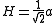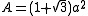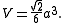## Other square pyramids

Other square pyramids have isosceles triangle sides.

For square pyramids in general, with base length l and height h, the surface area and volume are: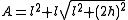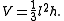## Related polyhedra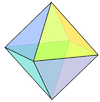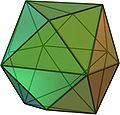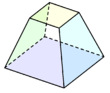A regular octahedron
Octahedron
In geometry, an octahedron is a polyhedron with eight faces. A regular octahedron is a Platonic solid composed of eight equilateral triangles, four of which meet at each vertex....

can be considered a square bipyramid
Bipyramid
An n-gonal bipyramid or dipyramid is a polyhedron formed by joining an n-gonal pyramid and its mirror image base-to-base.The referenced n-gon in the name of the bipyramids is not an external face but an internal one, existing on the primary symmetry plane which connects the two pyramid halves.The...

, i.e. two Johnson square pyramids connected base-to-base.
The tetrakis hexahedron
Tetrakis hexahedron
In geometry, a tetrakis hexahedron is a Catalan solid. Its dual is the truncated octahedron, an Archimedean solid. It can be seen as a cube with square pyramids covering each square face; that is, it is the Kleetope of the cube....

can be constructed from a cube
Cube
In geometry, a cube is a three-dimensional solid object bounded by six square faces, facets or sides, with three meeting at each vertex. The cube can also be called a regular hexahedron and is one of the five Platonic solids. It is a special kind of square prism, of rectangular parallelepiped and...

with short square pyramids added to each face.
Square frustum
Frustum
In geometry, a frustum is the portion of a solid that lies between two parallel planes cutting it....

is a square pyramid with the apex truncated.

### Dual polyhedron

The square pyramid is topologically a self-dual polyhedron. The dual edge lengths are different due to the polar reciprocation.
Dual Square pyramid Net of dual

## Topology

Like all pyramids, the square pyramid is self-dual, having the same number of vertices as faces.

A square pyramid can be represented by the Wheel graph
Wheel graph
In the mathematical discipline of graph theory, a wheel graph Wn is a graph with n vertices, formed by connecting a single vertex to all vertices of an -cycle. The numerical notation for wheels is used inconsistently in the literature: some authors instead use n to refer to the length of the cycle,...

W5.